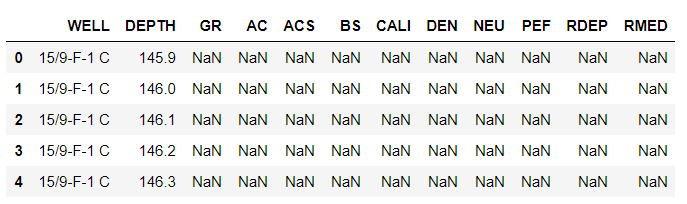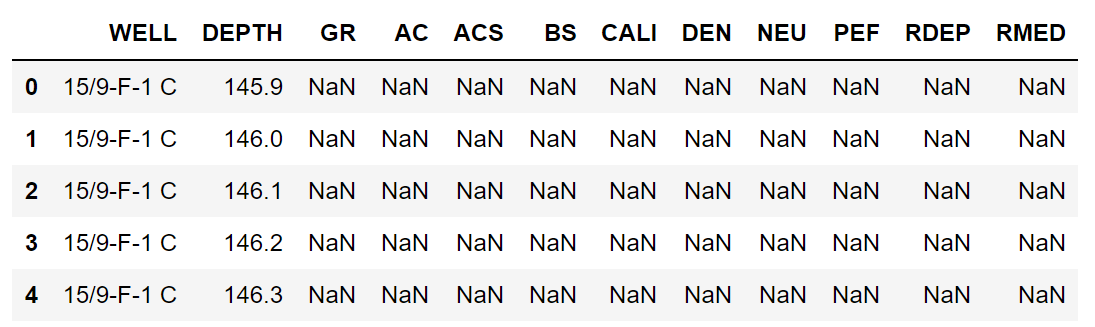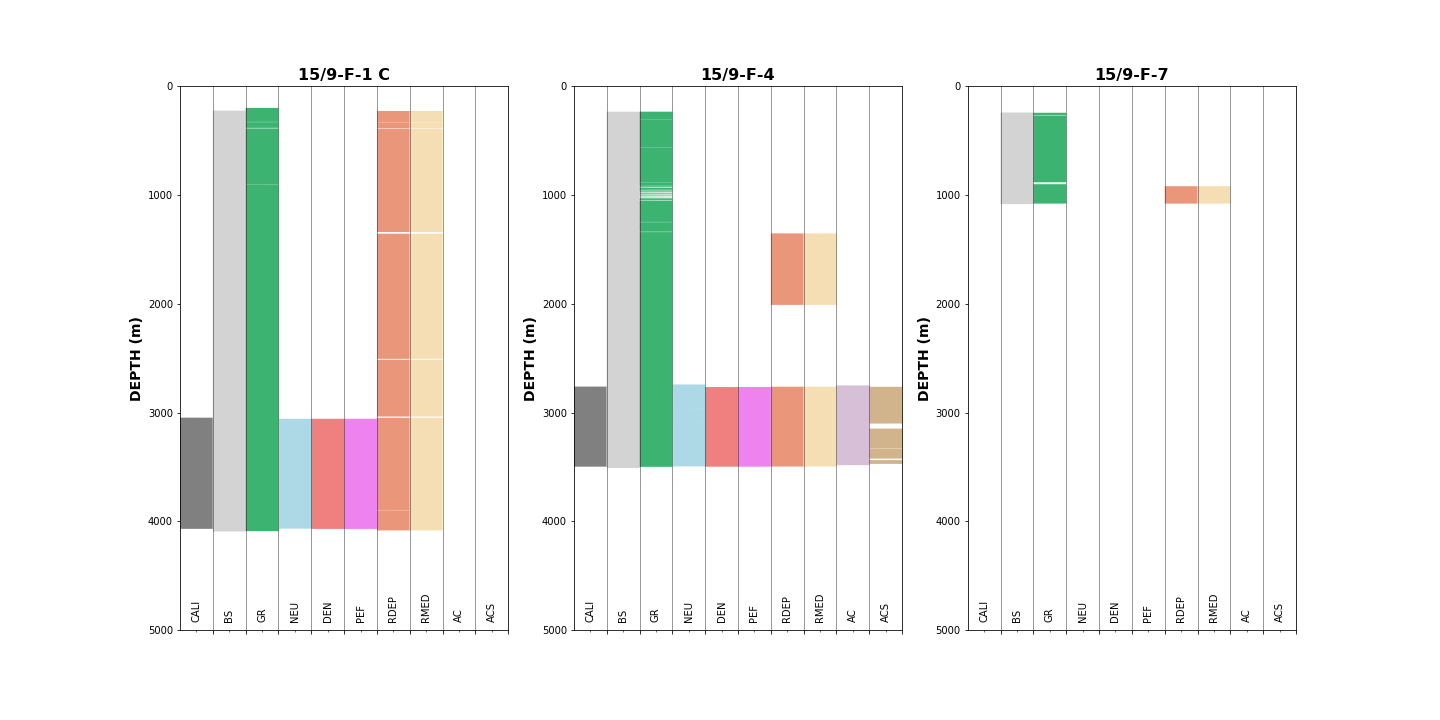## Visualising Well Data Coverage Using matplotlib

Exploratory Data Analysis (EDA) is an integral part of Data Science. The same is true for the petrophysical domain and can often be referred to as the Log QC or data review stage of a project. It is at this stage that we begin to go through the data in detail and identify what data we really have, where we have it and what is the quality of the gathered data.

A significant portion of the time that we spend (in some cases up to 90%! — Kohlleffel, 2015) working with well log data is spent trying to understand it and wrangle it into a state that is fit for interpretation. The remaining 10% is when we can get down to the business of carrying out the petrophysical interpretation. This can vary depending on the initial state of the project being worked on.

At the QC stage, we often we find ourselves with multiple input files, random curve names, missing data and extra curves that have no immediate use. This can lead to confusion and frustration, especially when working with multiple tools and vintage datasets. In cases where we have missing data, we need to identify it and determine the best way to handle it. This can be difficult to do by looking at single LAS files in a text editor, but it can be made easier using software. One such method is by using Python, a common and popular programming language.

In this short article, we will go over how to visualise data coverage within 3 wells of the Volve dataset that was released by Equinor in 2018.

You can find the full Jupyter Notebook for this example within 9 **— Visualising Data Covere.ipynb **of my Petrophysics and Python Series on GitHub at the following link: andymcdgeo/Petrophysics-Python-Series *This series of Jupyter Notebooks take you through various aspects of working with Python and Petrophysical data.*github.com

As with any Python project we need to load in the required data and libraries. For this article, we will be using pandas and matplotlib.

``````    import pandas as pd
import matplotlib.pyplot as plt

# Load in our data from a CSV file
``````

This will return:From a first glance, we can see that we have 12 columns in our dataset. The first column is the Well, followed by the Depth curve, which is subsequently followed by each of the logging curves.

To identify what wells we have in our dataset we could call data[‘WELL’].unique() which will return an array, but we can get a nicer format by implementing a short for loop and printing out each value.

``````    for well in data[‘WELL’].unique():
print(well)
``````

This will return a list of 3 wells:

• 15/9-F-1 C

• 15/9-F-4

• 15/9-F-7

## Data Preparation

In order for our plot to work as intended, we need to modify the column order of our dataset. This can be achieved by first creating a list of the columns in the order that we want.

``````    plot_cols = ['WELL', 'DEPTH', 'CALI', 'BS', 'GR', 'NEU', 'DEN', 'PEF', 'RDEP', 'RMED', 'AC', 'ACS']
``````

Then we can replace the existing dataframe with the new column order:

``````    data = data[plot_cols]
``````

We can then call upon data.head() to view the new dataframe.The next step involves creating a copy of our dataframe. This will allow us to keep the original dataframe for further work later in a project.

``````    data_nan = data.copy()
``````

In order for the plot to display as intended, we need to replace the values in the dataframe.

In places where we have a real value we will assign it a number which will be dependent on its position in the dataframe. In places where we have a NaN (Not a Number) value, we are going to give it a value of number - 1. This will allow us to shade between one number and another whilst using a single subplot for each well. This keeps things simple and negates the need for creating subplots for each curve in each well.

``````    for num, col in enumerate(data_nan.columns[2:]):
data_nan[col] = data_nan[col].notnull() * (num + 1)
data_nan[col].replace(0, num, inplace=True)
``````

Breaking down the above piece of code:

• `for num, col in enumerate(data_nan.columns[2:]:` This line is going to loop through each column from column number 2 onwards. enumerate() allows us to create a counter/index value as we loop through each column

• `data_nan[col] = data_nan[col].notnull()*(num+1)` Here we are converting the real values to a boolean (True or False). This is then converted to the column number plus 1 when true. When it is false, the values will be set to 0.

• `data_nan[col].replace(0, num, inplace=True)` We can now replace any 0 values with the column number.

## Plotting the Data

Now we have come to the plotting stage. In order for each well to plot in a separate subplot, we have to group the dataframe by the well name:

``````    grouped = data_nan.groupby('WELL')
``````

To plot the data we can call upon this short piece of code. I have added short comments to describe what each part is doing.

We use `ax.fillbetweenx()` to fill between our two values for each curve that we set up earlier. For example, CALI has two values to indicate data presence: 1 when there is a real value and 0 when there is a NaN. Similarly, GR has two values: 3 when there is real data and 2 when there is a NaN.

``````    #Setup the labels we want to display on the x-axis
labels = ['CALI', 'BS', 'GR', 'NEU', 'DEN', 'PEF', 'RDEP', 'RMED', 'AC', 'ACS']

#Setup the figure and the subplots
fig, axs = plt.subplots(1, 3, figsize=(20,10))

#Loop through each well and column in the grouped dataframe
for (name, df), ax in zip(grouped, axs.flat):
ax.set_xlim(0,9)

#Setup the depth range
ax.set_ylim(5000, 0)

#Create multiple fill betweens for each curve# This is between
# the number representing null values and the number representing
# actual values

ax.fill_betweenx(df.DEPTH, 0, df.CALI, facecolor='grey')
ax.fill_betweenx(df.DEPTH, 1, df.BS, facecolor='lightgrey')
ax.fill_betweenx(df.DEPTH, 2, df.GR, facecolor='mediumseagreen')
ax.fill_betweenx(df.DEPTH, 3, df.NEU, facecolor='lightblue')
ax.fill_betweenx(df.DEPTH, 4, df.DEN, facecolor='lightcoral')
ax.fill_betweenx(df.DEPTH, 5, df.PEF, facecolor='violet')
ax.fill_betweenx(df.DEPTH, 6, df.RDEP, facecolor='darksalmon')
ax.fill_betweenx(df.DEPTH, 7, df.RMED, facecolor='wheat')
ax.fill_betweenx(df.DEPTH, 8, df.AC, facecolor='thistle')
ax.fill_betweenx(df.DEPTH, 9, df.ACS, facecolor='tan')

#Setup the grid, axis labels and ticks
ax.grid(axis='x', alpha=0.5, color='black')
ax.set_ylabel('DEPTH (m)', fontsize=14, fontweight='bold')

#Position vertical lines at the boundaries between the bars
ax.set_xticks([1,2,3,4,5,6,7,8,9,10], minor=False)

#Position the curve names in the centre of each column
ax.set_xticks([0.5, 1.5 ,2.5 ,3.5 ,4.5 ,5.5 ,6.5 , 7.5, 8.5, 9.5], minor=True)

#Setup the x-axis tick labels
ax.set_xticklabels(labels,  rotation='vertical', minor=True, verticalalignment='bottom')
ax.set_xticklabels('', minor=False)

#Assign the well name as the title to each subplot
ax.set_title(name, fontsize=16, fontweight='bold')

plt.tight_layout()
plt.show()
``````

Once we run the code above, we get a matplotlib plot with 3 subplots. Each containing the data extent per well.From this plot we can determine:

15/9-F-1 C

• Minor gaps in the gamma ray and resistivity curves. As the gaps appear at same position on both resistivity curves we can make an initial assumption that they may be related to casing shoes. Further investigation would be needed to confirm this.

• Nuclear curves (DEN, NEU, PEF) and the caliper are only run over a short section, possibly indicating the zone of interest.

• No acoustic curves (AC and ACS)

15/9-F-4

• Contains all available curves, with the majority over a small section towards the bottom of the well.

• There are multiple gaps in the gamma ray (GR) and acoustic shear (ACS) curves. Could be tool related. Further investigation would reveal the cause.

15/9-F-7

• Minimal amount of data present over a short and shallow section.

• Only bitsize, gamma ray and resistivity measurements presents.

• Could potentially be caused by a tool failure or issues encountered whilst drilling. This information could be confirmed by reviewing the End of Well Reports, if they are available.

## Conclusion

In this short article, I have shown you how to load in data from a CSV file and visualise it in a way that you can identify where missing values are located. Understanding where you have or do not have data is a key step in exploring your dataset prior to carrying out a petrophysical interpretation or applying a machine learning model

Data used in this example: Equinor. (2018). Disclosing all Volve data. Available at: https://www.equinor.com/en/news/14jun2018-disclosing-volve-data.html

Share on: